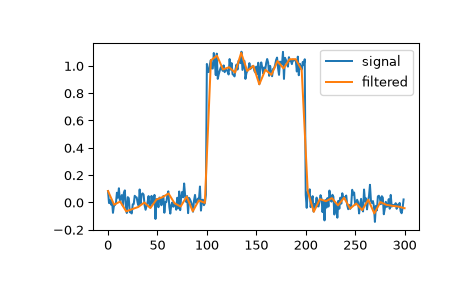# scipy.signal.qspline1d¶

scipy.signal.qspline1d(signal, lamb=0.0)[source]

Compute quadratic spline coefficients for rank-1 array.

Parameters
signalndarray

A rank-1 array representing samples of a signal.

lambfloat, optional

Smoothing coefficient (must be zero for now).

Returns
cndarray

`qspline1d_eval`

Evaluate a quadratic spline at the new set of points.

Notes

Find the quadratic spline coefficients for a 1-D signal assuming mirror-symmetric boundary conditions. To obtain the signal back from the spline representation mirror-symmetric-convolve these coefficients with a length 3 FIR window [1.0, 6.0, 1.0]/ 8.0 .

Examples

We can filter a signal to reduce and smooth out high-frequency noise with a quadratic spline:

```>>> import matplotlib.pyplot as plt
>>> from scipy.signal import qspline1d, qspline1d_eval
>>> rng = np.random.default_rng()
>>> sig = np.repeat([0., 1., 0.], 100)
>>> sig += rng.standard_normal(len(sig))*0.05  # add noise
>>> time = np.linspace(0, len(sig))
>>> filtered = qspline1d_eval(qspline1d(sig), time)
>>> plt.plot(sig, label="signal")
>>> plt.plot(time, filtered, label="filtered")
>>> plt.legend()
>>> plt.show()
```